# Five-Pointed Star

 < Day Day Up >

Follow these steps to make a five-pointed star:

1.

Draw a perfectly horizontal line. Then copy and paste it.

##### Figure A.15.2.

You'll first make a pentagon (which has five sides). To begin, with the duplicate line selected, use the Transform panel to rotate the line 72 degrees. You can figure the inside angle of any shape by dividing 360 (degrees) by the number of lines in the shape. In the case of a pentagon, each inside angle is 72 degrees because 360/5 = 72.

##### Figure A.16.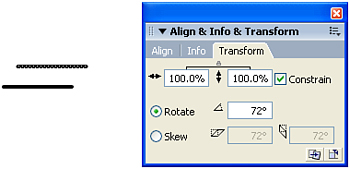3.

Select the line. Then click and drag the end of the line so that it snaps to the end of the first line.

##### Figure A.17.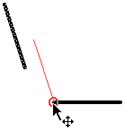4.

Duplicate the new line.

##### Figure A.18.5.

Rotate the new duplicate line by 72 degrees and snap it as you did before.

##### Figure A.19.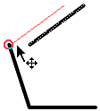6.

Repeat steps 3 5 to make a pentagon.

##### Figure A.20.7.

Draw a line inside the pentagon that doesn't touch any edges.

##### Figure A.21.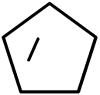8.

Grab one end point of the new line and snap it to the bottom-left corner of the pentagon.

##### Figure A.22.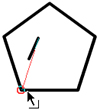9.

Grab the other end point and snap it to the top corner of the pentagon.

##### Figure A.23.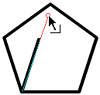10.

Draw another line in the larger area of the pentagon and connect it to the bottom-right and top corners of the pentagon.

##### Figure A.24.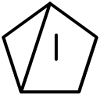11.

After you connect this new line, draw a small line in the space shown in the following figure and snap one end point to the bottom-right corner of the pentagon.

##### Figure A.25.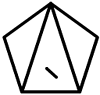12.

In one motion, drag the other end point to the middle-left corner of the pentagon. If you drag this line partway and stop, it will break where it crosses the other line.

##### Figure A.26.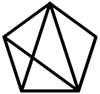13.

Draw another line in the space shown in the following figure.

##### Figure A.27.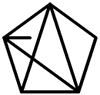14.

Snap the ends of the new line to the middle-left and middle-right corners of the pentagon. As in step 12, if you drag the line partway and stop before snapping it to the corner, the line will break.

##### Figure A.28.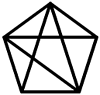15.

Draw the last line in the space shown in the following figure:

##### Figure A.29.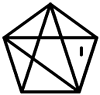16.

Snap this new line to the middle-right and bottom-left corners of the pentagon.

##### Figure A.30.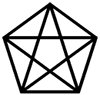17.

Remove excess lines.

##### Figure A.31.< Day Day Up >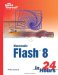Sams Teach Yourself Macromedia Flash 8 in 24 Hours
ISBN: 0672327546
EAN: 2147483647
Year: 2006
Pages: 235

Similar book on Amazon